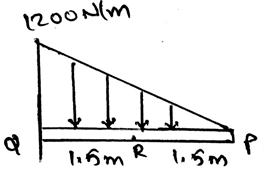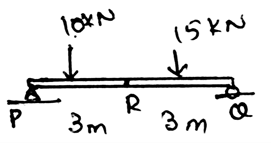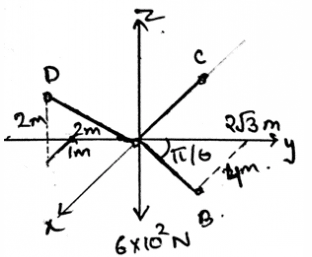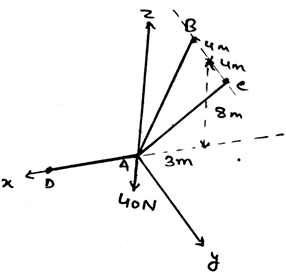Courses

# Test: Internal Forces Developed In Structural Members

## 30 Questions MCQ Test Engineering Mechanics | Test: Internal Forces Developed In Structural Members

Description
This mock test of Test: Internal Forces Developed In Structural Members for Mechanical Engineering helps you for every Mechanical Engineering entrance exam. This contains 30 Multiple Choice Questions for Mechanical Engineering Test: Internal Forces Developed In Structural Members (mcq) to study with solutions a complete question bank. The solved questions answers in this Test: Internal Forces Developed In Structural Members quiz give you a good mix of easy questions and tough questions. Mechanical Engineering students definitely take this Test: Internal Forces Developed In Structural Members exercise for a better result in the exam. You can find other Test: Internal Forces Developed In Structural Members extra questions, long questions & short questions for Mechanical Engineering on EduRev as well by searching above.
QUESTION: 1

### The determination of the internal loading is usually done so as to _________________

Solution:

The determination of the internal forces in the beam is done so as to design the beams as in the application purpose the beams will be subjected to many loads. This will help us to make the beam properly. And also this will ensure that the beams will not break after the loading is done on them.

QUESTION: 2

### The internal loading can be found by _________________

Solution:

The determination of the internal forces is done by various methods among which the method of section is the one. Also we have method of joints, which is done so as to determine the internal forces which are being developed in the trusses or the frames. This is done as to design them.

QUESTION: 3

### In method of sections we probably use the joints and take out the forces in the beams.

Solution:

In method of sections the determination of the forces is done over the trusses or the beams. It is not done on the joints. But yes the joints are used as the forces acting on the joints are used in the calculations. This is again used for the designing of the beams.

QUESTION: 4

The ____________ forces are used for the making of the free body diagram of the beams so as to apply the method of sections.

Solution:

The free body diagram is using the external forces which are acting on the beam as the main purpose is to design the beam. Design so as to withstand the loads which are going to be added to the beams. Thus the loads which are being added externally are being used in the free body diagrams.

QUESTION: 5

Rotational moments are not counted in the free body diagrams and the evaluation of the internal forces by the method of sections.

Solution:

In method of sections the determination of the forces is done over the trusses or the beams. It is not done on the joints. But yes the joints are used as the forces acting on the joints are used in the calculations. This is again used for the designing of the beams.

QUESTION: 6

The force acting perpendicular to the beam is called _______________ force.

Solution:

The forces which are acting perpendicular to the body of the beams are called as the normal forces. These are the forces which are supporting the beam. These forces are being calculated by the help of equilibrium equations. Thus the forces which are perpendicular to the surface are the normal forces.

QUESTION: 7

The force acting parallel/tangential to the beam is called _______________ force.

Solution:

The forces which are acting tangentially to the body of the beams are called as the shear forces. These are the forces which are giving moment to the beams. These forces are being calculated by the help of equilibrium equations. Thus the forces which are parallel to the surface are the shear forces.

QUESTION: 8

The couple moment produced in the beams are called as ____________________

Solution:

The forces are making the body tend to rotate. This is in general making the body rotate or make generate the moment in the beams. This moment is the same thing as the couple moment. But in beams it is referred as bending moment. And is again used for the designing of beams.

QUESTION: 9

The force components prevents the beam from which of the following process?

Solution:

The force components are the forces which are the ones which prevents the beam from relative translation. This means that the shearing generated will be held strongly by the help of the forces generated. The main motto is to make the beam stable and don’t allow the relative translation occur.

QUESTION: 10

The couple moment prevents the beam from which of the following process?

Solution:

The couple moments are the moments which are the ones which prevents the beam from relative rotation. This means that the rotation generated will be held strongly by the help of the opposite moments generated. The main motto is to make the beam stable and don’t allow the relative rotation to occur.

QUESTION: 11

According to the _________ law of Newton the loadings at the sections must act opposite to the forces applied.

Solution:

As the law state that there is always a reaction to every action applied. Thus if the forces are applied at the section of the beams, i.e. the external forces than there must be reactive forces. And these reactive forces act in opposite directions of the forces which are being applied externally.

QUESTION: 12

For the determination of the forces at the supports which part is to be considered in the calculations?

Solution:

The determination is usually done in this manner only. The selection is done as by selecting the one having one unknown and two known forces. This will ensure that the unknown value will be found. And also we have less number of unknowns which are needed to be founded.

QUESTION: 13

What is not the condition for the equilibrium in the beams if considered a section on which the method of sections is applied?

Solution:

For the equilibrium in beams by considering any of the axis we have all the conditions true as, ∑Fx=0, ∑Fy=0 and ∑Fz=0. Also we have the summation of the forces equal to zero. Which is not a non-zero value. Thus the conditions for the equilibrium of the beams.

QUESTION: 14

Determine the moment at the point R of the beam shown.Solution:

The loading acting upward is considered to be positive. This is done as to decrease the time used for the calculations. If the method of sections is being applied at the complex sections for the determination of the unknown forces, much time is required for it. Thus the main motto is to make the calculations easy and make the less use of time.

QUESTION: 15

Determine the normal force generated.Solution:

The loading acting upward is considered to be positive. This is done as to decrease the time used for the calculations. If the method of sections is being applied at the complex sections for the determination of the unknown forces, much time is required for it. Thus the main motto is to make the calculations easy and make the less use of time.

QUESTION: 16

The ____________ forces are used are used in the method of sections for the calculation of the internal forces.

Solution:

The calculations of the method of sections involve the making of the free diagrams and then use external forces to determine the internal forces. Design so as to withstand the loads which are going to be added to the beams. Thus the loads which are being added externally are being used in the free body diagrams.

QUESTION: 17

Every point on the force vector which is the internal force, is having the same magnitude and the same direction as the whole force vector have.

Solution:

The vector is made by joining the final and the starting point of the internal force. If we consider any arbitrary point then the length would be calculated w.r.t the starting point, which might give different magnitude but same direction. Thus the answer is false.

QUESTION: 18

For getting the normal force on the supports, we do what?

Solution:

The making of the vertical force sum zero makes the normal force to be determined. This means that the equilibrium equations when applied at the support gives us the answer. Hence the determination of the normal force is done easily by equating the vertical force sum equal to zero.

QUESTION: 19

For getting the horizontal component of the support reactions what do we do?

Solution:

The making of the horizontal force sum zero makes the horizontal force to be determined. This means that the equilibrium equations when applied at the support gives us the answer. Hence the determination of the horizontal force is done easily by equating the horizontal force sum equal to zero.

QUESTION: 20

Twisting moment is also called as __________

Solution:

The twisting moment is applied at the point where the couple moment is being applied or is made by the external forces. This makes the formation of the twisting moment along the beam, or the torsional moment. Thus the name twisting or the torsional moment.

QUESTION: 21

The loading generally act upon the ___________ of the body.

Solution:

The loads generally are applied on the centroid of the body. The moment of the body is also calculated along the centroid axis, thus the forces which are acting externally are always acting upon the centroid of the body. Gravity too is acted upon the centroid of the body.

QUESTION: 22

The area of does make the difference in the internal forces, that is if the area is large the internal force acting is also large and vice versa.

Solution:

This is because the internal forces are independent of the area. They are applied irrespective of the area given to the beams. They are dependent on the external forces. The more the external forces, the more are the internal force. That is the more is the amount of the internal forces.

QUESTION: 23

The magnitude of each loading will be ___________ at various points along the axis of the member of the beam.

Solution:

The magnitude of each loading will be different on the different points of the beams. The method of section is used to determine these forces. These forces are used so as to make the beams. That is the designing of the beams, the more are the forces than the stronger material is used for the making of the beams.

QUESTION: 24

Torsional moment is applied at the ___________ part of the beam.

Solution:

The torsional moment as said is made to be drawn at the centre of the beam. As it is generated by the various external forces, thus the centroid is the main point of the action of the various forces. Thus the forces which are made to be fallen on the body is having its effect over the centroid of the beam.

QUESTION: 25

Normal force is equal to _______________

Solution:

The normal force is determined by equating the vertical forces equal to zero. This makes the vertical forces to go on the other side of the equation. Suppose the L.H.S. is having the sum of the vertical forces then the R.H.S. is having the term normal force. That is the normal force is being equated to the negative of the sum of vertical forces.

QUESTION: 26

If the normal force creates a tension then the force is said to be ____________

Solution:

If the normal forces are giving tension to the body of the beam then the forces are said to be positive. That is if the normal force is making the tension to develop in the beam then it is considered to be positive. This is the basic sign convection that is being used to make the calculations easy.

QUESTION: 27

If the shear force creates a clockwise rotation then the force is said to be ____________

Solution:

If the shear forces are giving clockwise rotation to the body of the beam then the forces are said to be positive. That is if the shear force is making the beam to develop in the forces that tend to rotate it clockwise then it is considered to be positive. This is the basic sign convection that is being used to make the calculations easy.

QUESTION: 28

If the bending of the beam is concave upwards then the bending moment developed is called __________ moment.

Solution:

If the bending moment is giving concave bending upward to the body of the beam then the moment are said to be positive. That is if the bending moment is making the beam to develop in the forces that tend to bend the beam concave upward then it is considered to be positive. This is the basic sign convection that is being used to make the calculations easy.

QUESTION: 29

In the diagram given below, coordinates of D is (1, -2, 2), C (-2, 0, 0) and B are as shown. The dark region is the cables holding the weight of 600N at origin. Find the tension in the AD section.Solution:

As the system is in equilibrium so we need to balance the forces. So when apply the condition of net force to be zero in the z direction, we get (2/3)FAD = 600N. This gives us force along AD be 900N.

QUESTION: 30

Find the tension in the cable AC.Solution:

First represent the forces in their vector form. Then equate the net sum of the forces in the x, y and z directions to be zero. You will get FB = FC and 2(.848) = 40N. This gives the answer as 23.6N.